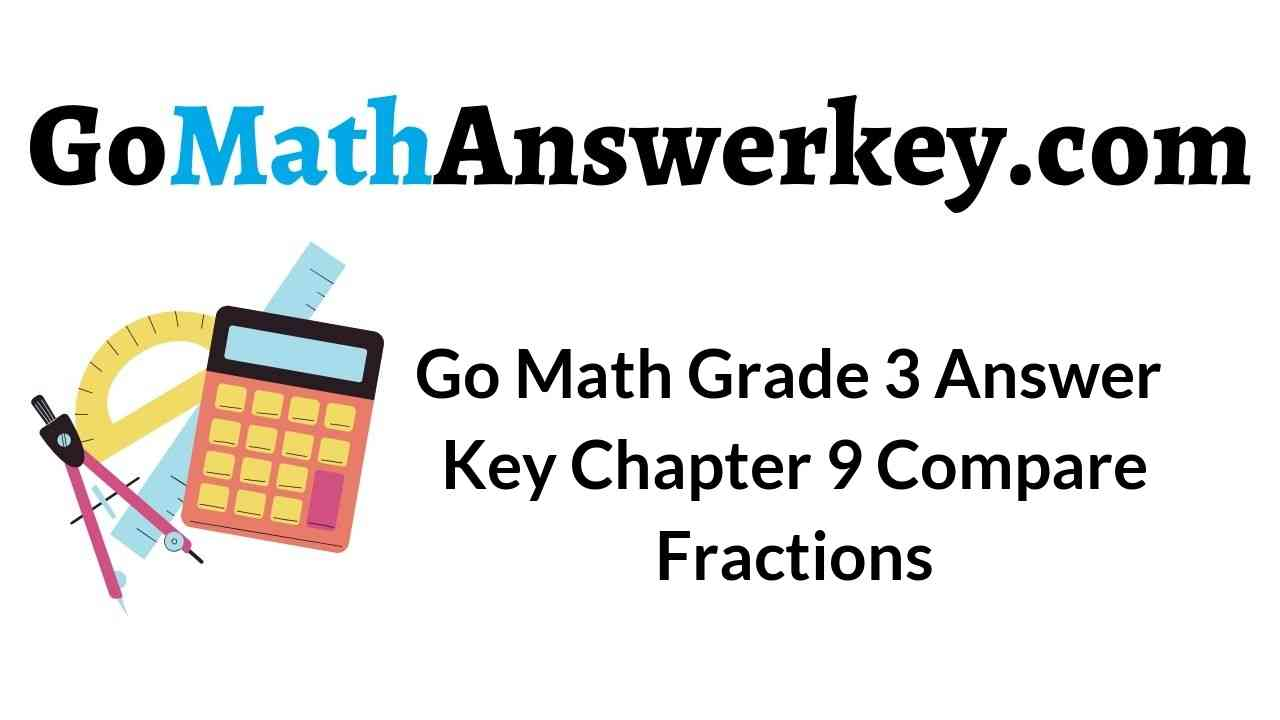# Go Math Grade 3 Chapter 9 Answer Key Pdf Compare Fractions

Go Math Grade 3 Chapter 9 Answer Key Pdf: In order to solve real-world mathematical problems, students must understand how the information is related, and analyze the relationships and draw conclusions. This will be possible only when you start from the basics. Download Free Pdf of Go Math Grade 3 Answer Key Chapter 9 Compare Fractions to practice the exercise and homework problems. Write the Mid Chapter Checkpoint test to know how much you learned previously from this chapter.

## Compare Fractions Go Math Grade 3 Chapter 9 Answer Key Pdf

The topics included in this chapter are Compare fractions with the same Numerator and Denominators, Equivalent Fractions, Compare and order fractions, and so on. Most of the students feel that fractions are difficult. Don’t worry we have provided the easy way to understand the concept of fractions. The HMH Go Math Grade 3 Answer Key Chapter 9 Compare Fractions helps your child to score the highest marks in the marks. So, practice the problems given in the 3rd Grade Go Math Solution Key Chapter 9 and try to solve the questions provided at the end of the chapter.

Lesson 1: Compare Fractions

Lesson 2: Problem Solving • Compare Fractions

Lesson 3: Compare Fractions with the Same Denominator

Lesson 4: Compare Fractions with the Same Numerator

Lesson 5: Compare Fractions

Mid -Chapter Checkpoint

Lesson 6: Compare and Order Fractions

Lesson 7: Model Equivalent Fractions

Lesson 9.7 – Page No. 548

Lesson 9: Equivalent Fractions

Review/ Test

### Compare Fractions – Page No. 509

Share and Show

Question 1.
At the park, people can climb a rope ladder to its top. Rosa climbed $$\frac{2}{8}$$ of the way up the ladder. Justin climbed $$\frac{2}{6}$$ of the way up the ladder. Who climbed higher on the rope ladder?
First, what are you asked to find?
Type below:
____________

Explanation:

Given,
Rosa climbed $$\frac{2}{8}$$ of the way up the ladder
Justin climbed $$\frac{2}{6}$$ of the way up the ladder
We are asked to find who climbed higher on the rope ladder
By comparing the denominators we can say that Justin Climbed higher than Rosa on the rope ladder.

Then, model and compare the fractions.
Type below:
____________Question 3.
Last, find the greater fraction.
$$\frac{2}{6}$$ _____ $$\frac{2}{8}$$

Answer: $$\frac{2}{6}$$ > $$\frac{2}{8}$$

Explanation:

When comparing fractions such as $$\frac{2}{8}$$ and $$\frac{2}{6}$$, you could also convert the fractions (if necessary) so they have the same denominator and then compare which numerator is larger.

Question 4.
___________ climbed higher on the rope ladder

Question 5.
What if Cara also tried the rope ladder and climbed $$\frac{2}{4}$$ of the way up? Who climbed the highest on the rope ladder: Rosa, Justin, or Cara? Explain how you know.
___________

Answer: If Cara also tried the rope ladder and climbed $$\frac{2}{4}$$ of the way up then Cara would have climbed highest on the rope ladder.
Because comparing fractions $$\frac{2}{4}$$, $$\frac{2}{6}$$, $$\frac{2}{8}$$ Cara climbed high among the three. The fraction $$\frac{2}{4}$$ is the greater than other 2 fractions. So by seeing this, we can say that Cara climbed highest on the rope ladder.

### Compare Fractions – Page No. 510

Use the table for 4–5.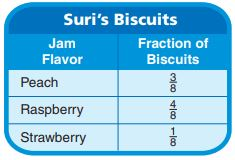Question 1.
Suri is spreading jam on 8 biscuits for breakfast. The table shows the fraction of biscuits spread with each jam flavor. Which flavor did Suri use on the most biscuits?
___________

Explanation:

The above table shows the fraction of jam frosted on the biscuits.
First, check the denominators to compare the fractions.
The denominators are the same. So, Compare with the numerators. The numerator of Raspberry is larger than other two flavors. So, Suri used Raspberry flavor on the most biscuits.

Question 2.
What’s the Question? The answer is strawberry
Type below:
___________

Suri is spreading jam on 8 biscuits for breakfast. The table shows the fraction of biscuits spread with each jam flavor. She frosted $$\frac{3}{8}$$ of the biscuits with peach jam, $$\frac{4}{8}$$ with raspberry jam, and $$\frac{1}{8}$$ with strawberry jam. Which flavor of jam did Suri use least on the biscuits?

Question 3.
Suppose Suri had also used plum jam on the biscuits. She frosted $$\frac{1}{2}$$ of the biscuits with peach jam, $$\frac{1}{4}$$ with raspberry jam, $$\frac{1}{8}$$ with strawberry jam, and $$\frac{1}{8}$$ with plum jam. Which flavor of jam did Suri use on the most biscuits?
___________

Explanation:

The fraction of peach jam is greater than raspberry jam, strawberry jam, and plum jam.
So, the answer is the peach jam.

Question 4.
Ms. Gordon has many snack bar recipes. One recipe uses $$\frac{1}{3}$$ cup oatmeal, $$\frac{1}{4}$$ vcup of milk, and $$\frac{1}{2}$$ cup flour. Which ingredient will Ms. Gordon use the most of?
___________

$$\frac{1}{2}$$ > $$\frac{1}{3}$$ and $$\frac{1}{4}$$
So, by comparing fractions we can say that Ms. Gordon uses the most flour for snack bar recipes.

Question 5.
Rick lives $$\frac{4}{6}$$ mile from school. Noah lives $$\frac{3}{6}$$ mile from school. Use the fractions and symbols to show which distance is longer.$$\frac{4}{6}$$ _____ $$\frac{3}{6}$$

Answer: $$\frac{4}{6}$$ > $$\frac{3}{6}$$

Explanation:

First of all, compare the denominators. If the denominators are the same then check the numerators.
Here 4 is greater than 3. So, $$\frac{4}{6}$$ > $$\frac{3}{6}$$

### Problem Solving Compare Fractions – Page No. 511

Solve.

Question 1.
Luis skates $$\frac{2}{3}$$ mile from his home to school. Isabella skates $$\frac{2}{4}$$ mile to get to school. Who skates farther?
Think: Use fraction strips to act it out.
Luis

Explanation:

Given,
Luis skates $$\frac{2}{3}$$ mile from his home to school.
Isabella skates $$\frac{2}{4}$$ mile to get to school.
To find Who stakes farther we have to compare the fractions.
$$\frac{2}{3}$$, $$\frac{2}{4}$$
The numerator of both fractions is the same and the denominators are different.
So, first, make the denominators equal.
$$\frac{2}{3}$$ × $$\frac{4}{4}$$ = $$\frac{8}{12}$$
$$\frac{2}{4}$$ × $$\frac{3}{3}$$ = $$\frac{6}{12}$$
Now denominators are the same.
Compare fractions $$\frac{8}{12}$$ and $$\frac{6}{12}$$
8 is greater than 6. So, $$\frac{8}{12}$$ > $$\frac{6}{12}$$
Therefore Luis Skates farther to school.

3rd Grade Math Review Pdf Topic 9 Lesson 9.2 Answer Key Question 2.
Sandra makes a pizza. She puts mushrooms on $$\frac{2}{8}$$ of the pizza. She adds green peppers to $$\frac{5}{8}$$ of the pizza. Which topping covers more of the pizza?
___________

Explanation:

Sandra makes a pizza. She puts mushrooms on $$\frac{2}{8}$$ of the pizza.
She adds green peppers to $$\frac{5}{8}$$ of the pizza.
Compare the fractions of mushrooms and green peppers.
$$\frac{2}{8}$$, $$\frac{5}{8}$$
The denominators are the same. So compare the numerators.
2 is lesser than 5. Thus $$\frac{2}{8}$$ < $$\frac{5}{8}$$
Thus Green Peppers covers more of the pizza.

Question 3.
The jars of paint in the art room have different amounts of paint. The green paint jar is $$\frac{4}{8}$$ full. The purple paint jar is $$\frac{4}{6}$$ full. Which paint jar is less full?
The _______ paint jar

Explanation:

The jars of paint in the art room have different amounts of paint.
The green paint jar is $$\frac{4}{8}$$ full.
The purple paint jar is $$\frac{4}{6}$$ full.
The numerators of both the fractions are the same.
Compare the denominators of green paint and purple paint jars.
The denominator with the greatest number will be the smallest fraction.
Therefore $$\frac{4}{8}$$ < $$\frac{4}{6}$$
Thus green paint jar is less full.

Question 4.
Jan has a recipe for bread. She uses $$\frac{2}{3}$$ cup of flour and $$\frac{1}{3}$$ cup of chopped onion. Which ingredient does she use more of, flour or onion?
_______

Explanation:

Jan has a recipe for bread. She uses $$\frac{2}{3}$$ cup of flour and $$\frac{1}{3}$$ cup of chopped onion.
Compare the fraction of flour and onion.
The denominators of both the fractions are the same. So, compare the numerators.
2 is greater than 1. Thus $$\frac{2}{3}$$ > $$\frac{1}{3}$$
That means Jan used more flour for bread.

Question 5.
Edward walked $$\frac{3}{4}$$ mile from his home to the park. Then he walked $$\frac{2}{4}$$ mile from the park to the library. Which distance is shorter?
_______

Answer: $$\frac{2}{4}$$ mile

Explanation:

Edward walked $$\frac{3}{4}$$ mile from his home to the park.
Then he walked $$\frac{2}{4}$$ mile from the park to the library.
To find the shorter distance we have to compare the fractions of Edward from home to park and from park to library.
$$\frac{3}{4}$$, $$\frac{2}{4}$$
The denominators of both the fractions are the same.
So compare the numerators.
3 is greater than 2. Thus $$\frac{3}{4}$$ > $$\frac{2}{4}$$
Thus the distance from the park to the library is shorter.

### Problem Solving Compare Fractions – Page No. 512

Lesson Check

Question 1.
Ali and Jonah collect seashells in identical buckets. When they are finished, Ali’s bucket is $$\frac{2}{6}$$ full and Jonah’s bucket is $$\frac{3}{6}$$ full. Which of the following correctly compares the fractions?
Options:
a. $$\frac{2}{6}$$ = $$\frac{3}{6}$$
b. $$\frac{2}{6}$$ > $$\frac{3}{6}$$
c. $$\frac{3}{6}$$ < $$\frac{2}{6}$$
d. $$\frac{3}{6}$$ > $$\frac{2}{6}$$

Answer: $$\frac{3}{6}$$ > $$\frac{2}{6}$$

Explanation:

Given that, Ali and Jonah collect seashells in identical buckets.
When they are finished, Ali’s bucket is $$\frac{2}{6}$$ full and Jonah’s bucket is $$\frac{3}{6}$$ full
Compare fractions $$\frac{2}{6}$$ and $$\frac{3}{6}$$
We observe that the denominators are the same. So, compare the numerators of both the fractions.
3 is greater than 2.
Thus $$\frac{3}{6}$$ > $$\frac{2}{6}$$
So, the correct answer is option D.

Question 2.
Rosa paints a wall in her bedroom. She puts green paint on $$\frac{5}{8}$$ of the wall and blue paint on $$\frac{3}{8}$$ of the wall. Which of the following correctly compares the fractions?
Options:
a. $$\frac{5}{8}$$ > $$\frac{3}{8}$$
b. $$\frac{5}{8}$$ < $$\frac{3}{8}$$
c. $$\frac{3}{8}$$ > $$\frac{5}{8}$$
d. $$\frac{3}{8}$$ = $$\frac{5}{8}$$

Answer: $$\frac{5}{8}$$ > $$\frac{3}{8}$$

Explanation:

Given:
Rosa paints a wall in her bedroom.
She puts green paint on $$\frac{5}{8}$$ of the wall and blue paint on $$\frac{3}{8}$$ of the wall.
The denominators are the same so compare the numerators of both the fractions.
5 is greater than 3. So, $$\frac{5}{8}$$ > $$\frac{3}{8}$$
Thus the correct answer is option A.

Spiral Review

Question 3.
Dan divides a pie into eightths. How many equal parts are there?
Options:
a. 3
b. 6
c. 8
d. 10

Dan divides a pie into eightths.
Eighths are nothing but the names of the parts. Eighths is equal to 8.
So, the correct answer is option C.

Question 4.
Which shows equal parts?
a.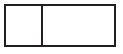b.c.d.Explanation:

Among all the figures circle is equally divided into 6 parts.
So, the answer is option B.

Go Math Lesson 9.2 Grade 3 Question 5.
Charles places 30 pictures on his bulletin board in 6 equal rows. How many pictures are in each row?
Options:
a. 3
b. 4
c. 5
d. 6

Explanation:

Given, Charles places 30 pictures on his bulletin board in 6 equal rows.
Number of pictures in each row = x
x × 6 = 30
x = 30/6 = 5
Therefore there are 5 pictures in each row.

Question 6.
Which of the following describes a pattern in the table?Options:
c. Multiply by 2.
d. Multiply by 5.

Explanation:

The above table shows that number of tables is multiplied by 5.
So, the correct answer is option D.

### Compare Fractions with the Same Denominator – Page No. 517

Compare. Write <, >, or =.

Question 1.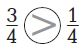Answer: $$\frac{3}{4}$$ > $$\frac{1}{4}$$

Explanation:

Check whether the denominators of the two fractions are the same.
Here the denominators of $$\frac{3}{4}$$ and $$\frac{1}{4}$$ are same. So compare the numerators.
3 is greater than 1.
Therefore, $$\frac{3}{4}$$ > $$\frac{1}{4}$$

Question 2.
$$\frac{3}{6}$$ ______ $$\frac{0}{6}$$

Answer: $$\frac{3}{6}$$ > $$\frac{0}{6}$$

Explanation:

First, check whether the denominators of the two fractions are the same or not.
After that compare the numerators.
3 > 0
So, $$\frac{3}{6}$$ > $$\frac{0}{6}$$

Question 3.
$$\frac{1}{2}$$ ______ $$\frac{1}{2}$$

Answer: $$\frac{1}{2}$$ = $$\frac{1}{2}$$

Explanation:

First, compare fractions with the same denominators.
If both are the same, then compare the numerators of both fractions.
The denominators and numerators are same for $$\frac{1}{2}$$
Thus $$\frac{1}{2}$$ = $$\frac{1}{2}$$

Question 4.
$$\frac{5}{6}$$ ______ $$\frac{6}{6}$$

Answer: $$\frac{5}{6}$$ < $$\frac{6}{6}$$

Explanation:

Compare the denominators of the fractions
The denominators of $$\frac{5}{6}$$ and $$\frac{6}{6}$$
Now compare the numerators of the fractions.
5 < 6
So, $$\frac{5}{6}$$ < $$\frac{6}{6}$$

$$\frac{7}{8}$$ ______ $$\frac{5}{8}$$

Answer: $$\frac{7}{8}$$ > $$\frac{5}{8}$$

Explanation:

Check whether the denominators are the same or not.
The denominator of $$\frac{7}{8}$$ and $$\frac{5}{8}$$ are same.
Now check the numerators 7 > 5.
Thus $$\frac{7}{8}$$ > $$\frac{5}{8}$$

Question 6.
$$\frac{2}{3}$$ ______ $$\frac{2}{3}$$

Answer: $$\frac{2}{3}$$ = $$\frac{2}{3}$$

Explanation:

Compare the denominators of 2 fractions.
Here numerators and denominators are the same.
So, $$\frac{2}{3}$$ = $$\frac{2}{3}$$

Question 7.
$$\frac{8}{8}$$ ______ $$\frac{0}{8}$$

Answer: $$\frac{8}{8}$$ > $$\frac{0}{8}$$

Explanation:

Check whether the denominators are the same. Now compare the numerators of two fractions.
8 > 0.
So, $$\frac{8}{8}$$ > $$\frac{0}{8}$$

Question 8.
$$\frac{1}{6}$$ ______ $$\frac{1}{6}$$

Answer: $$\frac{1}{6}$$ = $$\frac{1}{6}$$

Explanation:

When the denominators are the same, the whole is divided into the same size pieces. Now compare the numerators of both the fractions.
$$\frac{1}{6}$$ = $$\frac{1}{6}$$

Question 9.
$$\frac{3}{4}$$ ______ $$\frac{2}{4}$$

Answer: $$\frac{3}{4}$$ > $$\frac{2}{4}$$

Explanation:

Check whether the denominators are the same. If same that means the whole is divided into the same size pieces.
The denominators of $$\frac{3}{4}$$ and $$\frac{2}{4}$$
Now compare the numerators of both fractions.
3 is greater than 2.
Thus $$\frac{3}{4}$$ > $$\frac{2}{4}$$

Question 10.
$$\frac{1}{6}$$ ______ $$\frac{2}{6}$$

Answer: $$\frac{1}{6}$$ < $$\frac{2}{6}$$

Explanation:

Check whether the denominators are the same. If same that means the whole is divided into the same size pieces.
The denominators of $$\frac{1}{6}$$ and $$\frac{2}{6}$$
Now check the numerator 1 is less than 2.
Therefore, $$\frac{1}{6}$$ < $$\frac{2}{6}$$

Question 11.
$$\frac{1}{2}$$ ______ $$\frac{0}{2}$$

Answer: $$\frac{1}{2}$$ > $$\frac{0}{2}$$

Explanation:

When the denominators are the same, the whole is divided into the same pieces. Now check the numerators.
1 is greater than 0
Thus $$\frac{1}{2}$$ > $$\frac{0}{2}$$

Question 12.
$$\frac{3}{8}$$ ______ $$\frac{3}{8}$$

Answer: $$\frac{3}{8}$$ = $$\frac{3}{8}$$

Explanation:

Check the denominators of two fractions. If both are equal then compare the numerators.
The numerators of both fractions are equal.
So, $$\frac{3}{8}$$ = $$\frac{3}{8}$$

Question 13.
$$\frac{1}{4}$$ ______ $$\frac{4}{4}$$

Answer: $$\frac{1}{4}$$ < $$\frac{4}{4}$$

Explanation:

Compare fractions with the same denominators.
The denominators of $$\frac{1}{4}$$ and $$\frac{4}{4}$$ are same.
Compare numerators 1 and 4.
1 is less than 4.
Thus $$\frac{1}{4}$$ < $$\frac{4}{4}$$

Question 14.
$$\frac{5}{8}$$ ______ $$\frac{4}{8}$$

Answer: $$\frac{5}{8}$$ > $$\frac{4}{8}$$

Explanation:

Check whether the denominators are the same or not.
Now compare the numerators.
5 is greater than 4.
$$\frac{5}{8}$$ > $$\frac{4}{8}$$

Question 15.
$$\frac{4}{6}$$ ______ $$\frac{6}{6}$$

Answer: $$\frac{4}{6}$$ < $$\frac{6}{6}$$

Explanation:

Check whether the denominators of both the fractions are the same or not.
The denominators of $$\frac{4}{6}$$ and $$\frac{6}{6}$$ are the same.
Now compare the numerators 4 and 6.
4 is less than 6. So, $$\frac{4}{6}$$ < $$\frac{6}{6}$$

Problem Solving

Question 16.
Ben mowed $$\frac{5}{6}$$ of his lawn in one hour. John mowed $$\frac{4}{6}$$ of his lawn in one hour. Who mowed less of his lawn in one hour?
___________

Explanation:

Given,
Ben mowed $$\frac{5}{6}$$ of his lawn in one hour.
John mowed $$\frac{4}{6}$$ of his lawn in one hour.
Compare the denominators of both the fractions.
As the denominators are the same compare the numerators of the fractions.
4 is less than 5.
$$\frac{4}{6}$$ < $$\frac{5}{6}$$
So, John mowed less of his lawn in one hour.

Chapter 9 Go Math Grade 3 Question 17.
Darcy baked 8 muffins. She put blueberries in $$\frac{5}{8}$$ of the muffins. She put raspberries in $$\frac{3}{8}$$ of the muffins. Did more muffins have blueberries or raspberries?
___________

Explanation:

Given,
Darcy baked 8 muffins.
She put blueberries in $$\frac{5}{8}$$ of the muffins.
She put raspberries in $$\frac{3}{8}$$ of the muffins.
$$\frac{5}{8}$$ is greater than $$\frac{3}{8}$$
Thus there are more blueberries in muffins.

### Compare Fractions with the Same Denominator – Page No. 518

Lesson Check

Question 1.
Julia paints $$\frac{2}{6}$$ of a wall in her room white. She paints more of the wall green. Which fraction could show the part of the wall that is green?
Options:
a. $$\frac{1}{6}$$
b. $$\frac{2}{6}$$
c. $$\frac{3}{6}$$
d. $$\frac{0}{6}$$

Answer: $$\frac{3}{6}$$

Explanation:

First compare the denominators of given options and then compare the numerators
A. $$\frac{3}{6}$$ < $$\frac{2}{6}$$
B. $$\frac{2}{6}$$ = $$\frac{2}{6}$$
C. $$\frac{3}{6}$$ > $$\frac{2}{6}$$
D. $$\frac{0}{6}$$ < $$\frac{2}{6}$$
So, the answer is option C.

Question 2.
Liam is comparing fraction circles. Which of the following statements is true?
Options:
a. $$\frac{1}{2}$$ = $$\frac{1}{2}$$
b. $$\frac{3}{4}$$ > $$\frac{4}{4}$$
c. $$\frac{4}{6}$$ < $$\frac{3}{6}$$
d. $$\frac{2}{8}$$ = $$\frac{3}{8}$$

Answer: $$\frac{1}{2}$$ = $$\frac{1}{2}$$

Explanation:

Check whether the denominators are same. If both are same then compare the numerators.
$$\frac{1}{2}$$ = $$\frac{1}{2}$$
Option A is the correct answer.

Spiral Review

Question 3.
Mr. Edwards buys 2 new knobs for each of his kitchen cabinets. The kitchen has 9 cabinets. How many knobs does he buy?
Options:
a. 20
b. 18
c. 16
d. 12

Explanation:

Given, Mr. Edwards buys 2 new knobs for each of his kitchen cabinets.
The kitchen has 9 cabinets.
Number of knobs he buys = x
x = 9 × 2 = 18
Thus the correct answer is option B.

Question 4.
Allie builds a new bookcase with 8 shelves. She can put 30 books on each shelf. How many books can the bookcase hold?
Options:
a. 30
b. 38
c. 240
d. 300

Explanation:

Given that Allie builds a new bookcase with 8 shelves.
She can put 30 books on each shelf.
Let the number of books can the bookcase hold = y
y = 30 × 8
y = 240
Thus the correct answer is option C.

Question 5.
The Good Morning Café has 28 customers for breakfast. There are 4 people sitting at each table. How many tables are filled?
Options:
a. 8
b. 7
c. 6
d. 4

Explanation:

The Good Morning Café has 28 customers for breakfast.
There are 4 people sitting at each table.
Number of tables be t
t ×4 = 28
t = 28/4 = 4
Thus the number of tables filled = 7

Question 6.
Ella wants to use the Commutative Property of Multiplication to help find the product 5 × 4. Which number sentence can she use?
Options:
a. 5 + 4 = 10
b. 5 × 5 = 25
c. 5 − 4 = 1
d. 4 × 5 = 20

Answer: 4 × 5 = 20

Explanation:

According to the commutative property of multiplication, changing the order of the numbers we are multiplying, does not change the product.
a × b = b × a
5 × 4 = 4 × 5 = 20
So, the correct answer is option D.

### Compare Fractions with the Same Numerator – Page No. 523

Compare. Write <, >, or =.

Question 1.Question 2.
$$\frac{3}{8}$$ ______ $$\frac{3}{6}$$

Answer: $$\frac{3}{8}$$ < $$\frac{3}{6}$$

Explanation:

When comparing fractions with the same numerator, the fraction with the smaller denominator is greater.
So, $$\frac{3}{8}$$ < $$\frac{3}{6}$$

Question 3.
$$\frac{2}{3}$$ ______ $$\frac{2}{4}$$

Answer: $$\frac{2}{3}$$ > $$\frac{2}{4}$$

Explanation:

Compare the fractions $$\frac{2}{3}$$ and $$\frac{2}{4}$$
The numerators of both the fractions are the same. So compare the denominators.
The fraction with the smaller denominator is greater
So, $$\frac{2}{3}$$ > $$\frac{2}{4}$$

Question 4.
$$\frac{2}{8}$$ ______ $$\frac{2}{3}$$

Answer: $$\frac{2}{8}$$ < $$\frac{2}{3}$$

Explanation:

Compare $$\frac{2}{8}$$ and $$\frac{2}{3}$$
When comparing fractions with the same numerator, the fraction with the smaller denominator is greater.
$$\frac{2}{8}$$ < $$\frac{2}{3}$$

Question 5.
$$\frac{3}{6}$$ ______ $$\frac{3}{4}$$

Answer: $$\frac{3}{6}$$ < $$\frac{3}{4}$$

Explanation:

Compare the fractions $$\frac{3}{6}$$ and $$\frac{3}{4}$$
The numerators are the same and the denominators are different.
The number with the smallest number will be the greatest.
So, $$\frac{3}{6}$$ < $$\frac{3}{4}$$

Question 6.
$$\frac{1}{2}$$ ______ $$\frac{1}{6}$$

Answer: $$\frac{1}{2}$$ > $$\frac{1}{6}$$

Explanation:

When comparing fractions with the same numerator, the fraction with the smaller denominator is greater.
2 is greater than 6.
$$\frac{1}{2}$$ > $$\frac{1}{6}$$

Go Math Grade 3 Chapter 9 Pdf Lesson 9.4 Compare Fractions Question 7.
$$\frac{5}{6}$$ ______ $$\frac{5}{8}$$

Answer: $$\frac{5}{6}$$ > $$\frac{5}{8}$$

Explanation:

We observe that numerators are the same and the denominators are different.
The fraction with the smallest number will be the greatest.
So, $$\frac{5}{6}$$ > $$\frac{5}{8}$$

Question 8.
$$\frac{4}{8}$$ ______ $$\frac{4}{8}$$

Answer: $$\frac{4}{8}$$ = $$\frac{4}{8}$$

Explanation:

The numerators and denominators of both the fractions are the same.
So, $$\frac{4}{8}$$ = $$\frac{4}{8}$$

Question 9.
$$\frac{6}{8}$$ ______ $$\frac{6}{6}$$

Answer: $$\frac{6}{8}$$ < $$\frac{6}{6}$$

Explanation:

Compare the fractions $$\frac{6}{8}$$ and $$\frac{6}{6}$$
We observe that numerators are the same and the denominators are different.
So, $$\frac{6}{8}$$ < $$\frac{6}{6}$$

Problem Solving

Question 10.
Javier is buying food in the lunch line. The tray of salad plates is $$\frac{3}{8}$$ full. The tray of fruit plates is $$\frac{3}{4}$$ full. Which tray is more full?
The tray of ______ plates

Explanation:

Javier is buying food in the lunch line. The tray of salad plates is $$\frac{3}{8}$$ full.
The tray of fruit plates is $$\frac{3}{4}$$ full.
Compare the fraction of salad plates and fruit plates.
$$\frac{3}{8}$$ and $$\frac{3}{4}$$
The numerators are the same. So compare the denominators.
So, $$\frac{3}{8}$$ <$$\frac{3}{4}$$
Thus the fruit plate tray is more full than the salad plate tray.

Question 11.
Rachel bought some buttons. Of the buttons, $$\frac{2}{4}$$ are yellow and $$\frac{2}{8}$$ are red. Rachel bought more of which color buttons?
More _______ buttons

Explanation:

Rachel bought some buttons. Of the buttons, $$\frac{2}{4}$$ are yellow and $$\frac{2}{8}$$ are red.
Compare $$\frac{2}{4}$$ and $$\frac{2}{8}$$
The fraction with the smaller denominator is greater.
$$\frac{2}{4}$$ >$$\frac{2}{8}$$
Therefore there are more yellow buttons.

### Compare Fractions with the Same Numerator – Page No. 524

Lesson Check

Question 1.
Which symbol makes the statement true?
$$\frac{3}{4}$$ O $$\frac{3}{8}$$
a. >
b. <
c. =
d. none

Explanation:

In the above statement, the fractions are of the same numerators. So, we need to check the denominators.
The number with the highest number will be the least fraction.
So, $$\frac{3}{4}$$ > $$\frac{3}{8}$$
Thus the correct answer is option A.

Question 2.
Which symbol makes the statement true?
$$\frac{2}{4}$$ O $$\frac{2}{3}$$
a. >
b. <
c. =
d. none

Explanation:

The fractions are of the same numerators. So, we need to see the denominators.
The number with the highest number will be the least fraction.
So, $$\frac{2}{4}$$ < $$\frac{2}{3}$$
So, the correct answer is option B.

Spiral Review

Question 3.
Anita divided a circle into 6 equal parts and shaded 1 of the parts. Which fraction names the part she shaded?
Options:
a. $$\frac{1}{6}$$
b. $$\frac{1}{5}$$
c. $$\frac{5}{6}$$
d. $$\frac{1}{1}$$

Answer: $$\frac{1}{6}$$

Explanation:

Given that, Anita divided a circle into 6 equal parts and shaded 1 of the parts.
So, the fraction of the shaded part is $$\frac{1}{6}$$
Thus the correct answer is option A.

Question 4.
Which fraction names the shaded part of the rectangle?Options:
a. $$\frac{1}{8}$$
b. $$\frac{2}{8}$$
c. $$\frac{6}{8}$$
d. $$\frac{8}{8}$$

Answer: $$\frac{2}{8}$$

Explanation:

The rectangle is divided into 8 equal parts. Out of 8 two parts are shaded.
So, the fraction name of the shaded part is $$\frac{2}{8}$$
The correct answer is option B.

Question 5.
Chip worked at the animal shelter for 6 hours each week for several weeks. He worked for a total of 42 hours. Which of the following can be used to find the number of weeks Chip worked at the animal shelter?
Options:
a. 6 + 42
b. 42 − 6
c. 42 ÷ 6
d. 42 × 6

Explanation:

Chip worked at the animal shelter for 6 hours each week for several weeks.
Number of hours he worked = 42 hours
Number of weeks he worked at the animal shelter = x
x × 6 = 42
x = 42 ÷ 6
Thus the correct answer is option C.

Question 6.
Mr. Jackson has 20 quarters. If he gives 4 quarters to each of his children, how many children does Mr. Jackson have?
Options:
a. 3
b. 4
c. 5
d. 6

Explanation:

Given, Mr. Jackson has 20 quarters.
If he gives 4 quarters to each of his children
Number of children Mr. Jackson have = y
y × 4 = 20
y = 20/4 = 5
Therefore, Mr. Jackson has 5 children.

### Compare Fractions – Page No. 529

Compare. Write <, >, or =. Write the strategy you used.

Question 1.Question 2.
$$\frac{2}{3}$$ ______ $$\frac{7}{8}$$

Answer: $$\frac{2}{3}$$ < $$\frac{7}{8}$$

Missing pieces

Explanation:

Compare the fractions $$\frac{2}{3}$$, $$\frac{7}{8}$$
The numerators and denominators are different here.
$$\frac{2}{3}$$ × $$\frac{8}{8}$$ = $$\frac{16}{24}$$
$$\frac{7}{8}$$ × $$\frac{3}{3}$$ = $$\frac{21}{24}$$
16 is less than 24.
So, $$\frac{16}{24}$$ < $$\frac{21}{24}$$
That means $$\frac{2}{3}$$ < $$\frac{7}{8}$$

Question 3.
$$\frac{3}{4}$$ ______ $$\frac{1}{4}$$

Answer: $$\frac{3}{4}$$ > $$\frac{1}{4}$$

Explanation:

The Denominator is the same here. So compare the numerators.
$$\frac{3}{4}$$, $$\frac{1}{4}$$
3 is greater than 1.
Thus $$\frac{3}{4}$$ > $$\frac{1}{4}$$

Name a fraction that is less than or greater than the given fraction. Draw to justify your answer.

Go Math Grade 3 Chapter 9 Operations with Fractions Answer Key Question 4.
greater than $$\frac{1}{3}$$
Type below:
___________

Answer: $$\frac{2}{3}$$

Explanation: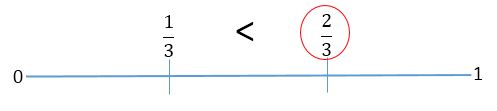$$\frac{2}{3}$$ is greater than $$\frac{1}{3}$$

Question 5.
less than $$\frac{3}{4}$$
Type below:
___________

Answer: $$\frac{2}{4}$$

Explanation: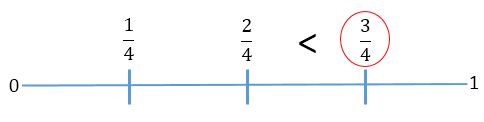$$\frac{2}{4}$$ is less than $$\frac{3}{4}$$

Problem Solving

Question 6.
At the third-grade party, two groups each had their own pizza. The blue group ate $$\frac{7}{8}$$ pizza. The green group ate $$\frac{2}{8}$$ pizza. Which group ate more of their pizza?
The _______ group

Explanation:

Given,
The blue group ate $$\frac{7}{8}$$ pizza.
The green group ate $$\frac{2}{8}$$ pizza.
Compare the fractions of the blue group and green group.
$$\frac{7}{8}$$ and $$\frac{2}{8}$$
The denominators are the same here. So compare the numerators.
The numerator with the greatest number will be the greatest fraction.
Therefore $$\frac{7}{8}$$ > $$\frac{2}{8}$$
So, the blue group ate more pizza.

Question 7.
Ben and Antonio both take the same bus to school. Ben’s ride is $$\frac{7}{8}$$ mile. Antonio’s ride is $$\frac{3}{4}$$ mile. Who has a longer bus ride?
___________

Explanation:

Ben and Antonio both take the same bus to school.
Ben’s ride is $$\frac{7}{8}$$ mile.
Antonio’s ride is $$\frac{3}{4}$$ mile.
Compare the fractions $$\frac{7}{8}$$, $$\frac{3}{4}$$
Make the denominators equal to compare the fractions.
$$\frac{3}{4}$$ × $$\frac{8}{8}$$ = $$\frac{24}{32}$$
$$\frac{7}{8}$$ × $$\frac{4}{4}$$ = $$\frac{28}{32}$$
$$\frac{28}{32}$$ > $$\frac{24}{32}$$
$$\frac{7}{8}$$ > $$\frac{3}{4}$$
Thus Ben has a longer bus ride.

### Compare Fractions – Page No. 530

Lesson Check

Question 1.
Which statement is correct?
Options:
a. $$\frac{2}{3}$$ > $$\frac{7}{8}$$
b. $$\frac{2}{3}$$ < $$\frac{7}{8}$$
c. $$\frac{2}{3}$$ = $$\frac{7}{8}$$
d. $$\frac{7}{8}$$ = $$\frac{2}{3}$$

Answer: $$\frac{2}{3}$$ < $$\frac{7}{8}$$

Explanation:

A. $$\frac{2}{3}$$ > $$\frac{7}{8}$$
Here the numerator of one fraction is greater than the numerator of the other fraction.
So, $$\frac{2}{3}$$ is not greater than $$\frac{7}{8}$$
B. $$\frac{2}{3}$$ < $$\frac{7}{8}$$
Here the numerator of one fraction is greater than the numerator of the other fraction.
2 is less than 7.
Thus the statement $$\frac{2}{3}$$ < $$\frac{7}{8}$$ is true.

Question 2.
Which symbol makes the statement true?
$$\frac{2}{4}$$ O $$\frac{2}{6}$$
a. >
b. <
c. =
d. none

Explanation:

Compare the fractions $$\frac{2}{4}$$ & $$\frac{2}{6}$$
The numerator of both fractions is the same.
So we need to compare the denominators.
The denominator with the greater number will be the smallest fraction.
Therefore, $$\frac{2}{4}$$ > $$\frac{2}{6}$$
The correct answer is option A.

Spiral Review

Question 3.
Cam, Stella, and Rose each picked 40 apples. They put all their apples in one crate. How many apples are in the crate?
Options:
a. 40
b. 43
c. 120
d. 123

Explanation:

Given that, Cam, Stella, and Rose each picked 40 apples.
They put all their apples in one crate.
That means each person picked 40 apples = 40 + 40 + 40 = 120 apples
Therefore there are 120 apples in the crate.
The correct answer is option C.

Question 4.
Each shape is 1 whole. Which fraction is represented by the shaded part of the model?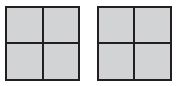Options:
a. $$\frac{2}{4}$$
b. $$\frac{4}{4}$$
c. $$\frac{8}{4}$$
d. $$\frac{8}{1}$$

Answer: $$\frac{8}{4}$$

Explanation:

From the figure we observe that there are 2 squares.
Each square is divided into 4 equal parts.
Total number of shaded parts = 4
So, the fraction of 1 whole shaded part is $$\frac{8}{4}$$
Thus the correct answer is option C.

Question 5.
Which related multiplication fact can you use to find
16 ÷ ■ = 2?
Options:
a. 4 × 4 = 16
b. 8 × 2 = 16
c. 8 × 1 = 8
d. 4 × 2 = 8

Answer: 8 × 2 = 16

Explanation:

16 ÷ ■ = 2
■ = 16/2 = 8
So, the related multiplication fact of 16 ÷ ■ = 2 is 8 × 2 = 16.
The correct answer is option B.

Question 6,
What is the unknown factor?
9 × ■ = 36
Options:
a. 7
b. 6
c. 4
d. 3

Explanation:

■ is the unknown factor
9 × ■ = 36
■ = 36/9 = 4
Thus the correct answer is option C.

### Mid -Chapter Checkpoint – Page No. 531

Concepts and Skills

Question 1.
When two fractions refer to the same whole, explain why the fraction with a lesser denominator has larger pieces than the fraction with a greater denominator.
Type below:
___________

Answer: If two fractions have the same numerator but different denominators the fraction with greater denominator is smaller.
Example: Let us consider an apple that is divided into equal parts.
If the apple is divided among 2 people.
Then each get $$\frac{1}{2}$$ part of the apple.
If the apple is divided among 4 people.
Then each get $$\frac{1}{4}$$ part of an apple.
Hence, in the first case, people get more amount of apple than the second.
Hence, if as a whole the denominator is less the fraction is greater.

Question 2.
When two fractions refer to the same whole and have the same denominators, explain why you can compare only the numerators
Type below:
___________

Answer: If the denominators are the same, then the fraction with the greater numerator is the greater fraction. The fraction with the lesser numerator is the lesser fraction.
Example:
Let us consider a pizza which is cut into 4 parts.
One person ate 3 pieces then the fraction is $$\frac{3}{4}$$
And the other person ate 1 piece then the fraction is $$\frac{1}{4}$$
Now compare the fractions $$\frac{1}{4}$$ and $$\frac{3}{4}$$
First person ate 2 pieces more than the second person.
Hence the fraction with the greater numerator is the greater fraction.

Compare. Write <, >, or =.

Question 3.
$$\frac{1}{6}$$ ______ $$\frac{1}{4}$$

Answer: $$\frac{1}{6}$$ < $$\frac{1}{4}$$

Explanation:

$$\frac{1}{6}$$, $$\frac{1}{4}$$
In this case the numerators are same and the denominators are different.
We know that the two fractions have the same numerator but different denominators the fraction with greater denominator is smaller.
So, $$\frac{1}{6}$$ < $$\frac{1}{4}$$

Question 4.
$$\frac{1}{8}$$ ______ $$\frac{1}{8}$$

Answer: $$\frac{1}{8}$$ = $$\frac{1}{8}$$

Explanation:

Compare the fractions $$\frac{1}{8}$$ & $$\frac{1}{8}$$
The numerators and the denominators are the same here. Thus these are the equivalent fractions.
Hence, $$\frac{1}{8}$$ = $$\frac{1}{8}$$

Question 5.
$$\frac{2}{8}$$ ______ $$\frac{2}{3}$$

Answer: $$\frac{2}{8}$$ < $$\frac{2}{3}$$

Explanation:

Compare the fractions $$\frac{2}{8}$$ & $$\frac{2}{3}$$
The numerators are the same and the denominators are different.
As we know the denominators with the greatest number will be the smallest fraction.
$$\frac{2}{8}$$ < $$\frac{2}{3}$$

Question 6.
$$\frac{4}{2}$$ ______ $$\frac{1}{2}$$

Answer: $$\frac{4}{2}$$ > $$\frac{1}{2}$$

Explanation:

Compare both the fractions $$\frac{4}{2}$$ and $$\frac{1}{2}$$
In this case, the denominators are the same but the numerators are different.
So compare the numerators.
4 is greater than 1.
Thus $$\frac{4}{2}$$ > $$\frac{1}{2}$$

Question 7.
$$\frac{7}{8}$$ ______ $$\frac{3}{8}$$

Answer: $$\frac{7}{8}$$ > $$\frac{3}{8}$$

Explanation:

Comparing the fractions $$\frac{7}{8}$$ and $$\frac{3}{8}$$
The denominators are same but the numerators are different.
We know that the denominators are the same, then the fraction with the greater numerator is the greater fraction. The fraction with the lesser numerator is the lesser fraction.
7 is greater than 3.
So, $$\frac{7}{8}$$ > $$\frac{3}{8}$$

Question 8.
$$\frac{5}{6}$$ ______ $$\frac{2}{3}$$

Answer: $$\frac{5}{6}$$ > $$\frac{2}{3}$$

Explanation:

Compare the fractions $$\frac{5}{6}$$ and $$\frac{2}{3}$$
In this case the numerators and the denominators are different.
So, we have to make the denominators equal.
$$\frac{5}{6}$$ × $$\frac{3}{3}$$ = $$\frac{15}{18}$$
$$\frac{2}{3}$$ × $$\frac{6}{6}$$ = $$\frac{12}{18}$$
Now the denominators are same. So compare the numerators 15 is greater than 12.
So, $$\frac{15}{18}$$ > $$\frac{12}{18}$$
That means $$\frac{5}{6}$$ > $$\frac{2}{3}$$

Question 9.
$$\frac{2}{4}$$ ______ $$\frac{3}{4}$$

Answer: $$\frac{2}{4}$$ < $$\frac{3}{4}$$

Explanation:

The denominators are the same. So compare the fractions with the numerators.
2 is less than 3.
So, $$\frac{2}{4}$$ < $$\frac{3}{4}$$

Question 10.
$$\frac{6}{6}$$ ______ $$\frac{6}{8}$$

Answer: $$\frac{6}{6}$$ > $$\frac{6}{8}$$

Explanation:

Here the numerators are same but the denominators are different.
We know that denominators with the greatest number will be the smallest fraction.
Therefore, $$\frac{6}{6}$$ > $$\frac{6}{8}$$

Question 11.
$$\frac{3}{4}$$ ______ $$\frac{7}{8}$$

Answer: $$\frac{3}{4}$$ < $$\frac{7}{8}$$

Explanation:

Compare the fractions $$\frac{3}{4}$$ and $$\frac{7}{8}$$
The numerators and denominators are different.
So, we have to make the denominators equal.
$$\frac{3}{4}$$ × $$\frac{8}{8}$$ = $$\frac{24}{32}$$
$$\frac{7}{8}$$ × $$\frac{4}{4}$$ = $$\frac{28}{32}$$
Now the denominators are equal. So compare the numerators of both the fractions.
$$\frac{24}{32}$$ < $$\frac{28}{32}$$
Therefore $$\frac{3}{4}$$ < $$\frac{7}{8}$$

Name a fraction that is less than or greater than the given fraction. Draw to justify your answer.

Question 12.
greater than $$\frac{2}{6}$$
Type below:
___________

Answer: $$\frac{4}{6}$$
$$\frac{4}{6}$$ > $$\frac{2}{6}$$

Question 13.
less than $$\frac{2}{3}$$
Type below:
___________

Answer: $$\frac{1}{3}$$
$$\frac{1}{3}$$ < $$\frac{2}{3}$$

### Mid-Chapter Checkpoint – Page No. 532

Question 14.
Two walls in Tiffany’s room are the same size. Tiffany paints $$\frac{1}{4}$$ of one wall. Roberto paints $$\frac{1}{8}$$ of the other wall. Who painted a greater amount in Tiffany’s room?
___________

Explanation:

Given that, Two walls in Tiffany’s room are the same size. Tiffany paints $$\frac{1}{4}$$ of one wall.
Roberto paints $$\frac{1}{8}$$ of the other wall.
The numerators are the same and the denominators are different here.
So, we have to make the denominators same.
$$\frac{1}{4}$$ × $$\frac{8}{8}$$ = $$\frac{8}{32}$$
$$\frac{1}{8}$$ × $$\frac{4}{4}$$ = $$\frac{4}{32}$$
Now compare the fractions $$\frac{8}{32}$$ and $$\frac{4}{32}$$
8 is greater than 4.
$$\frac{8}{32}$$ > $$\frac{4}{32}$$
Therefore, Tiffany painted greater amount than Roberto.

Question 15.
Matthew ran $$\frac{5}{8}$$ mile during track practice. Pablo ran $$\frac{5}{6}$$ mile. Who ran farther?
___________

Explanation:

Given,
Matthew ran $$\frac{5}{8}$$ mile during track practice.
Pablo ran $$\frac{5}{6}$$ mile.
Compare the fractions $$\frac{5}{8}$$ and $$\frac{5}{6}$$
Numerators are the same and denominators are different.
The denominator with the greatest number will be the smallest fraction.
Thus $$\frac{5}{8}$$ < $$\frac{5}{6}$$
Pablo ran farther than Matthew.

Question 16.
Mallory bought 6 roses for her mother. Two-sixths of the roses are red and $$\frac{4}{6}$$ is yellow. Did Mallory buy fewer red roses or yellow roses?
___________

Explanation:

Mallory bought 6 roses for her mother.
Two-sixths of the roses are red and $$\frac{4}{6}$$ is yellow.
Compare fractions $$\frac{4}{6}$$ and $$\frac{2}{6}$$
The denominators of both the fractions are the same and numerators are different.
4 is greater than 2. So, $$\frac{4}{6}$$ > $$\frac{2}{6}$$
Therefore Mallory buys fewer red roses.

Question 17.
Lani used $$\frac{2}{3}$$ cup of raisins, $$\frac{3}{8}$$ cup of cranberries, and $$\frac{3}{4}$$ cup of oatmeal to bake cookies. Which ingredient did Lani use the least amount of?
___________

Explanation:

Lani used $$\frac{2}{3}$$ cup of raisins, $$\frac{3}{8}$$ cup of cranberries, and $$\frac{3}{4}$$ cup of oatmeal to bake cookies.
Compare the fractions $$\frac{2}{3}$$, $$\frac{3}{8}$$ and $$\frac{3}{4}$$
The numerators and denominators are different in this case.
The denominator with the highest number will be the smallest fraction.
Therefore Lani used the least amount of cranberries.

### Compare and Order Fractions – Page No. 537

Write the fractions in order from greatest to least.

Question 1.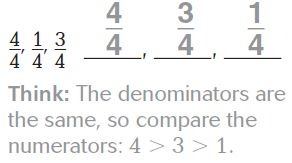Question 2.
$$\frac{2}{8}$$, $$\frac{5}{8}$$, $$\frac{1}{8}$$
Type below:
___________

Answer: $$\frac{5}{8}$$, $$\frac{2}{8}$$, $$\frac{1}{8}$$

Explanation:

The denominators of three fractions are same. So compare the numerators of the fractions.
5 is greater than 2 is greater than 1.
Thus the order of the fraction is $$\frac{5}{8}$$, $$\frac{2}{8}$$, $$\frac{1}{8}$$

Question 3.
$$\frac{1}{3}$$, $$\frac{1}{6}$$, $$\frac{1}{2}$$
Type below:
___________

Answer: $$\frac{1}{2}$$, $$\frac{1}{3}$$, $$\frac{1}{6}$$

Explanation:

Compare the three fractions $$\frac{1}{3}$$, $$\frac{1}{6}$$, $$\frac{1}{2}$$
We observe that the numerators of the fractions are same and the denominators are different.
We know that the denominators with the smallest number will be the greater fraction.
Therefore, $$\frac{1}{2}$$, $$\frac{1}{3}$$, $$\frac{1}{6}$$

Go Math 3rd Grade Pdf Model Equivalent Fractions Lesson 9.6 Question 4.
$$\frac{2}{3}$$, $$\frac{2}{6}$$, $$\frac{2}{8}$$
Type below:
___________

Answer: $$\frac{2}{3}$$, $$\frac{2}{6}$$, $$\frac{2}{8}$$

Explanation:

Comparing the fractions $$\frac{2}{3}$$, $$\frac{2}{6}$$, $$\frac{2}{8}$$
The numerators are the same but the denominators are different.
We know that the denominators with the greatest number will be the smallest fraction.
3 is greater than 6 is greater than 8.
So, the order of the fraction is $$\frac{2}{3}$$, $$\frac{2}{6}$$, $$\frac{2}{8}$$

Write the fractions in order from least to greatest.

Question 5.
$$\frac{2}{4}$$, $$\frac{4}{4}$$, $$\frac{3}{4}$$
Type below:
___________

Answer: $$\frac{2}{4}$$, $$\frac{3}{4}$$, $$\frac{4}{4}$$

Explanation:

The denominators are same but the numerators are different.
So compare the numerators of three fractions.
2 < 3 < 4
$$\frac{2}{4}$$, $$\frac{3}{4}$$, $$\frac{4}{4}$$

Question 6.
$$\frac{4}{6}$$, $$\frac{5}{6}$$, $$\frac{2}{6}$$
Type below:
___________

Answer: $$\frac{2}{6}$$, $$\frac{4}{6}$$, $$\frac{5}{6}$$

Explanation:

Compare the fractions $$\frac{4}{6}$$, $$\frac{5}{6}$$, $$\frac{2}{6}$$
The denominators are same but the numerators are different.
So compare the numerators of the three fractions.
The order of fractions from least to greatest is $$\frac{2}{6}$$, $$\frac{4}{6}$$, $$\frac{5}{6}$$

Question 7.
$$\frac{7}{8}$$, $$\frac{0}{8}$$, $$\frac{3}{8}$$
Type below:
___________

Answer: $$\frac{0}{8}$$, $$\frac{3}{8}$$, $$\frac{7}{8}$$

Explanation:

Compare the fractions $$\frac{7}{8}$$, $$\frac{0}{8}$$, $$\frac{3}{8}$$
The numerators of the fractions are different. But the denominators are same.
0 < 3 < 7
The order from least to greatest is $$\frac{0}{8}$$, $$\frac{3}{8}$$, $$\frac{7}{8}$$

Question 8.
$$\frac{3}{4}$$, $$\frac{3}{6}$$, $$\frac{3}{8}$$
Type below:
___________

Answer: $$\frac{3}{8}$$, $$\frac{3}{6}$$, $$\frac{3}{4}$$

Explanation:

Compare the fractions $$\frac{3}{4}$$, $$\frac{3}{6}$$, $$\frac{3}{8}$$
The numerators are same but the denominators of the three fractions are different.
So, compare the denominators.
The denominators with the greatest number will be the smallest fraction.
So, the order of fractions from least to greatest is $$\frac{3}{8}$$, $$\frac{3}{6}$$, $$\frac{3}{4}$$

Problem Solving

Question 9.
Mr. Jackson ran $$\frac{7}{8}$$ mile on Monday. He ran $$\frac{3}{8}$$ mile on Wednesday and $$\frac{5}{8}$$ mile on Friday. On which day did Mr. Jackson run the shortest distance?
On ___________

Explanation:

Mr. Jackson ran $$\frac{7}{8}$$ mile on Monday.
He ran $$\frac{3}{8}$$ mile on Wednesday and $$\frac{5}{8}$$ mile on Friday
The denominators of the fractions are the same. So, compare the numerators.
Compare to all Mr. Jackson run the shortest distance on Wednesday.

Question 10.
Delia has three pieces of ribbon. Her red ribbon is $$\frac{2}{4}$$ foot long. Her green ribbon is $$\frac{2}{3}$$ foot long. Her yellow ribbon is $$\frac{2}{6}$$ foot long. She wants to use the longest piece for a project. Which color ribbon should Delia use?
The _______ ribbon

Explanation:

Delia has three pieces of ribbon. Her red ribbon is $$\frac{2}{4}$$ foot long.
Her green ribbon is $$\frac{2}{3}$$ foot long.
Her yellow ribbon is $$\frac{2}{6}$$ foot long.
Compare the fractions to know which color should Delia use.
$$\frac{2}{4}$$, $$\frac{2}{3}$$ and $$\frac{2}{6}$$
The numerators of the three fractions are the same but the denominators are different.
$$\frac{2}{3}$$ is longest among all.
so, the answer is Green ribbon.

### Compare and Order Fractions – Page No. 538

Lesson Check

Question 1.
Which list orders the fractions from least to greatest?
Options:
a. $$\frac{1}{8}$$, $$\frac{1}{3}$$, $$\frac{1}{6}$$
b. $$\frac{1}{3}$$, $$\frac{1}{6}$$, $$\frac{1}{8}$$
c. $$\frac{1}{8}$$, $$\frac{1}{6}$$, $$\frac{1}{3}$$
d. $$\frac{1}{6}$$, $$\frac{1}{8}$$, $$\frac{1}{3}$$

Answer: $$\frac{1}{8}$$, $$\frac{1}{6}$$, $$\frac{1}{3}$$

Explanation:

When the numerators are the same, think about the denominators to compare and order fractions.
The denominator with the greatest number is the smallest fraction.
$$\frac{1}{8}$$ < $$\frac{1}{6}$$ < $$\frac{1}{3}$$
So, the order is $$\frac{1}{8}$$, $$\frac{1}{6}$$, $$\frac{1}{3}$$

Question 2.
Which list orders the fractions from greatest to least?
Options:
a. $$\frac{3}{8}$$, $$\frac{3}{6}$$, $$\frac{3}{4}$$
b. $$\frac{3}{4}$$, $$\frac{3}{6}$$, $$\frac{3}{8}$$
c. $$\frac{3}{4}$$, $$\frac{3}{8}$$, $$\frac{3}{4}$$
d. $$\frac{3}{6}$$, $$\frac{3}{4}$$, $$\frac{3}{8}$$

Answer: $$\frac{3}{4}$$, $$\frac{3}{6}$$, $$\frac{3}{8}$$

Explanation:

If the numerators are the same, think about the denominators to compare and order fractions.
The denominators with the smallest number will be the greatest fraction.
$$\frac{3}{4}$$ > $$\frac{3}{6}$$ > $$\frac{3}{8}$$
Thus the fractions from greatest to least are $$\frac{3}{4}$$, $$\frac{3}{6}$$, $$\frac{3}{8}$$

Spiral Review

Question 3.
What fraction of the group of cars is shaded?Options:
a. $$\frac{3}{8}$$
b. $$\frac{1}{2}$$
c. $$\frac{5}{8}$$
d. $$\frac{3}{5}$$

Answer: $$\frac{3}{8}$$

Explanation:

Total number of cars = 8
Number of shaded cars among those 8 cars = 3
So, the fraction of the shaded cars = 3/8
Thus the answer is option A.

Question 4.
Wendy has 6 pieces of fruit. Of these, 2 pieces are bananas. What fraction of Wendy’s fruit is bananas?
Options:
a. $$\frac{2}{6}$$
b. $$\frac{2}{4}$$
c. $$\frac{4}{6}$$
d. $$\frac{2}{2}$$

Answer: $$\frac{2}{6}$$

Explanation:

Given that, Wendy has 6 pieces of fruit.
Of these, 2 pieces are bananas.
The fraction of Wendy’s fruit is 2/6
Thus the correct answer is $$\frac{2}{6}$$ i.e., option A.

Go Math Grade 3 Lesson 6 Compare and Order Fractions Answer Key Question 5.
Toby collects data and makes a bar graph about his classmates’ pets. He finds that 9 classmates have dogs, 2 classmates have fish, 6 classmates have cats, and 3 classmates have gerbils. Which pet will have the longest bar on the bar graph?
Options:
a. dog
b. fish
c. cat
d. gerbil

Explanation:

Number of classmates who have dogs = 9
Number of classmates who have fish = 2
Number of classmates who have cats = 6
Number of classmates who have gerbils = 3
So, dogs will have the longest bar on the bar graph.

Question 6.
The number sentence is an example of which multiplication property?
6 × 7 = (6 × 5) + (6 × 2)
Options:
a. Associative
b. Commutative
c. Distributive
d. Identity

Explanation:

6 × 7 = (6 × 5) + (6 × 2)
Here 7 is distributed into 5 + 2
According to the distributive property, multiplying the sum of two or more addends by a number will give the same result as multiplying each addend individually by the number and then adding the products together.
So, the answer is option C.

### Model Equivalent Fractions – Page No. 543

Shade the model. Then divide the pieces to find the equivalent fraction.

Question 1.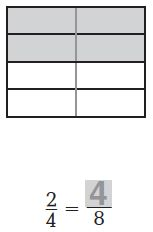Answer: $$\frac{4}{8}$$
Explanation:

The figure shows that there are 8 equal parts and 4 of them are shaded.
The Fraction of the shaded part is $$\frac{4}{8}$$
Thus, $$\frac{4}{8}$$ = $$\frac{2}{4}$$

Question 2.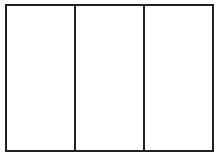$$\frac{1}{3}$$ = $$\frac{■}{6}$$
$$\frac{□}{□}$$

Answer: $$\frac{2}{6}$$

Explanation: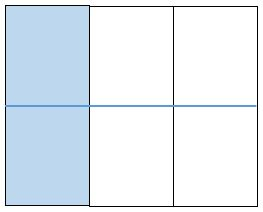There are 6 equal parts in which 2 parts are shaded. Now the fraction for the shaded part is $$\frac{2}{6}$$

$$\frac{1}{3}$$ = $$\frac{2}{6}$$

Use the number line to find the equivalent fraction.

Question 3.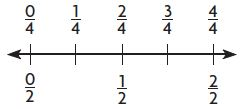$$\frac{1}{2}$$ = $$\frac{■}{4}$$
$$\frac{□}{□}$$

Answer: $$\frac{2}{4}$$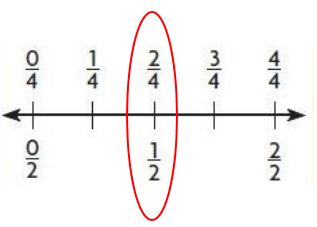The fraction $$\frac{1}{2}$$ and $$\frac{2}{4}$$ lies on the same point.

Therefore, $$\frac{1}{2}$$ = $$\frac{2}{4}$$$$\frac{3}{4}$$ = $$\frac{■}{8}$$
$$\frac{□}{□}$$

Answer: $$\frac{6}{8}$$The above figure shows that the point $$\frac{6}{8}$$ and $$\frac{3}{4}$$ lies on the same point on the number line.

Thus $$\frac{3}{4}$$ = $$\frac{6}{8}$$

Problem Solving

Question 5.
Mike says that $$\frac{3}{3}$$ of his fraction model is shaded blue. Ryan says that $$\frac{6}{6}$$ of the same model is shaded blue. Are the two fractions equivalent? If so, what is another equivalent fraction?
___________

Answer: $$\frac{2}{2}$$

Explanation:

Mike says that $$\frac{3}{3}$$ of his fraction model is shaded blue.
Ryan says that $$\frac{6}{6}$$ of the same model is shaded blue.
The two fractions are equivalent.
$$\frac{3}{3}$$ = $$\frac{6}{6}$$ = $$\frac{2}{2}$$

Question 6.
Brett shaded $$\frac{4}{8}$$ of a sheet of notebook paper. Aisha says he shaded $$\frac{1}{2}$$ of the paper. Are the two fractions equivalent? If so, what is another equivalent fraction?
___________

Answer: $$\frac{2}{4}$$

Explanation:

$$\frac{1}{2}$$ = $$\frac{4}{8}$$
So, the two fractions are equivalent.
The another equivalent fraction is $$\frac{2}{4}$$.

### Model Equivalent Fractions – Page No. 544

Lesson Check

Question 1.
Find the fraction equivalent to $$\frac{2}{3}$$.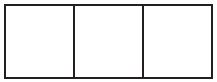Options:
a. $$\frac{3}{2}$$
b. $$\frac{4}{6}$$
c. $$\frac{3}{6}$$
d. $$\frac{1}{3}$$

Answer: $$\frac{4}{6}$$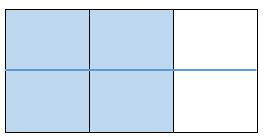$$\frac{2}{3}$$ = $$\frac{4}{6}$$

Go Math Grade 3 Chapter 9 Review Test Answer Key Question 2.
Find the fraction equivalent to $$\frac{1}{4}$$.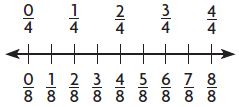Options:
a. $$\frac{1}{2}$$
b. $$\frac{2}{4}$$
c. $$\frac{2}{8}$$
d. $$\frac{6}{8}$$

Answer: $$\frac{2}{8}$$

Explanation:

The fugure shows that the $$\frac{1}{4}$$ and $$\frac{2}{8}$$ lies on the same point.
So, the equivalent fraction of $$\frac{1}{4}$$ is $$\frac{2}{8}$$
Thus the correct answer is option C.

Spiral Review

Question 3.
Eric practiced piano and guitar for a total of 8 hours this week. He practiced the piano for $$\frac{1}{4}$$ of that time. How many hours did Eric practice the piano this week?
Options:
a. 6 hours
b. 4 hours
c. 3 hours
d. 2 hours

Explanation:

Eric practiced piano and guitar for a total of 8 hours this week.
He practiced the piano for $$\frac{1}{4}$$ of that time.
To find how many hours did Eric practice the piano this week
You need to multiply the total number of hours with a fraction of the time he practiced.
8 ×  $$\frac{1}{4}$$ = 2 hours
Thus the correct answer is option D.

Question 4.
Kylee bought a pack of 12 cookies. One-third of the cookies are peanut butter. How many of the cookies in the pack are peanut butter?
Options:
a. 9
b. 6
c. 4
d. 3

Explanation:

Given,
Kylee bought a pack of 12 cookies.
One-third of the cookies are peanut butter.
To find the number of cookies in the pack is peanut butter.
Multiply number of cookies with a fraction of cookies are peanut butter
12 × $$\frac{1}{3}$$ = 4
So, the correct answer is option C.

Question 5.
There are 56 students going to the game. The coach puts 7 students in each van. Which number sentence can be used to find how many vans are needed to take the students to the game?
Options:
a. 56 + 7 = ■
b. ■ + 7 = 56
c. ■ × 7 = 56
d. 56 − 7 = ■

Answer: ■ × 7 = 56

Explanation:

There are 56 students going to the game.
The coach puts 7 students in each van.
Let ■ be the number of vans
56 ÷ 7 = ■
■ × 7 = 56
■ = 8
Thus the correct answer is option C.

Question 6.
Which number sentence can be used to describe the picture?Options:
a. 2 + 4 = 6
b. 4 − 2 = 2
c. 4 × 1 = 4
d. 8 ÷ 2 = 4

Answer: 8 ÷ 2 = 4

Explanation:

Number of counters = 8
Number of equal groups = 4
Number in each group = 2
The division equation is 8 ÷ 2 = 4
So, the answer is option D.

### Lesson 9.7 – Page No. 548

Question 13.
Christy bought 8 muffins. She chose 2 apple, 2 banana, and 4 blueberry. She and her family ate the apple and banana muffins for breakfast. What fraction of the muffins did they eat? Write an equivalent fraction. Draw a picture.
$$\frac{□}{□}$$

Answer: $$\frac{4}{8}$$

Explanation:

Given:
Christy bought 8 muffins.
She chose 2 apples, 2 bananas, and 4 blueberries. She and her family ate the apple and banana muffins for breakfast.
They had 2 apples and 2 banana muffins for their breakfast.
Only 4 blueberries are left out of 8 muffins.
The fraction of the muffins they ate = $$\frac{4}{8}$$ or $$\frac{1}{2}$$

Go Math Third Grade Pdf Chapter 9 Review Test Question 14.
After dinner, $$\frac{2}{3}$$ of the cornbread is left. Suppose 4 friends want to share it equally. What fraction names how much of the whole pan of cornbread each friend will get? Use the model on the right. Explain your answer.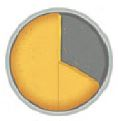$$\frac{□}{□}$$

Answer: $$\frac{1}{6}$$

Explanation:

To know the fraction of the whole pan of cornbread that each friend gets. I divide each third into 2 equal pieces to get 4 pieces in all.
$$\frac{2}{3}$$ = $$\frac{1}{6}$$ + $$\frac{1}{6}$$ + $$\frac{1}{6}$$ + $$\frac{1}{6}$$
That means each friend gets $$\frac{1}{6}$$ of cornbread of the whole pan.

Question 15.
There are 16 people having lunch. Each person wants $$\frac{1}{4}$$ of a pizza. How many whole pizzas are needed? Draw a picture to show your answer.
______ pizzas

Explanation:

Given that, There are 16 people having lunch.
Each person wants $$\frac{1}{4}$$ of a pizza.
Multiply the total number of people by a fraction of each pizza for one person.
= 16 × $$\frac{1}{4}$$
= 16/4 = 4
Therefore 4 pizzas are needed for 16 people.

Question 16.
Lucy has 5 oatmeal bars, each cut in half. What fraction names all of the oatmeal bar halves?$$\frac{□}{□}$$

Answer: $$\frac{10}{2}$$

Explanation:

Lucy has 5 oatmeal bars, each cut in half.
Total number of oatmeal bars = 5
5 oatmeal bars are divided into halves = 5 + 5 = 10
So. the fraction name for all of the oatmeal bar halves = $$\frac{10}{2}$$

Question 16.
What if Lucy cuts each part of the oatmeal bar into 2 equal pieces to share with friends? What fraction names all of the oatmeal bar pieces now?
$$\frac{□}{□}$$

Answer: $$\frac{10}{2}$$

Explanation:

The above figure shows that there are 5 oatmeal bars.
And each oatmeal bar is divided into halves = 5 × 2 = 10
The fraction for the all of the oatmeal bar halves = $$\frac{10}{2}$$
The equivalent fraction of $$\frac{10}{2}$$ is $$\frac{20}{4}$$

Question 17.
Mr. Peters made a pizza. There is $$\frac{4}{8}$$ of the pizza left over. Select the fractions that are equivalent to the part of the pizza that is left over. Mark all that apply.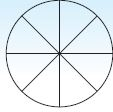Options:
a. $$\frac{5}{8}$$
b. $$\frac{3}{4}$$
c. $$\frac{2}{4}$$
d. $$\frac{1}{2}$$

Answer: $$\frac{2}{4}$$

Explanation:

Mr. Peters made a pizza. There is $$\frac{4}{8}$$ of the pizza left over.
The equivalent fraction of $$\frac{4}{8}$$ is $$\frac{2}{4}$$
So, the correct answer is option C.

### Equivalent Fractions – Page No. 549

Each shape is 1 whole. Shade the model to find the equivalent fraction.

Question 1.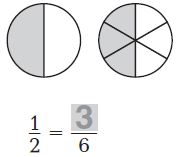Answer: $$\frac{3}{6}$$

Explanation:

The circle is divided into 6 equal groups. Each group is $$\frac{1}{6}$$ of the whole circle.
There are 3 shaded parts in the circle.
So, the fraction of the shaded part is $$\frac{3}{6}$$.
$$\frac{1}{2}$$ = $$\frac{3}{6}$$

Question 2.$$\frac{3}{4}$$ = $$\frac{6}{■}$$
$$\frac{□}{□}$$

Answer: $$\frac{6}{8}$$

Explanation:

The square is divided into 8 equal parts. Each group is $$\frac{1}{8}$$ of the whole square.
There are 6 shaded parts in the square.
Thus the fraction of the shaded part is 6/8
So, $$\frac{3}{4}$$ = $$\frac{6}{8}$$

Circle equal groups to find the equivalent fraction.

Question 3.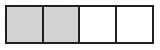$$\frac{2}{4}$$ = $$\frac{■}{2}$$
$$\frac{□}{□}$$

Answer: $$\frac{1}{2}$$

Explanation:

Rectangle is divided into 4 equal parts. The fraction of each group is 1/4.
There are 2 shaded parts out of 4 = $$\frac{2}{4}$$
latex]\frac{2}{4}[/latex] = $$\frac{1}{2}$$

Question 4$$\frac{4}{6}$$ = $$\frac{■}{3}$$
$$\frac{□}{□}$$

Answer: $$\frac{2}{3}$$

Explanation:

The figure shows that the rectangle is divided into 6 equal parts and 4 are shaded among them.
The fraction of the shaded part is $$\frac{4}{6}$$
We can also write it as $$\frac{2}{3}$$
Thus, $$\frac{4}{6}$$ = $$\frac{2}{3}$$

Problem Solving

Question 5.
May painted 4 out of 8 equal parts of a poster board blue. Jared painted 2 out of 4 equal parts of a same-size poster board red. Write fractions to show which part of the poster board each person painted.
Type below:
____________

Answer: May $$\frac{4}{8}$$; Jared $$\frac{2}{4}$$

Explanation:

Given that, May painted 4 out of 8 equal parts of a poster board blue.
4 parts are shaded out of 8 parts. So, the fraction of the shaded part is $$\frac{4}{8}$$
Jared painted 2 out of 4 equal parts of a same-size poster board red.
The fraction of the shaded part is $$\frac{2}{4}$$

Question 6.
Are the fractions equivalent? Draw a model to explain.
____________

### Equivalent Fractions – Page No. 550

Lesson Check

Question 1.
Which fraction is equivalent to $$\frac{6}{8}$$?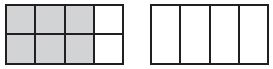Options:
a. $$\frac{1}{4}$$
b. $$\frac{1}{3}$$
c. $$\frac{3}{4}$$
d. $$\frac{4}{6}$$

Answer: $$\frac{3}{4}$$

Explanation: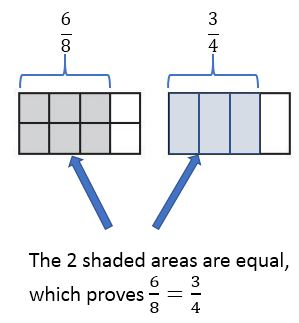$$\frac{6}{8}$$ = $$\frac{3}{4}$$
So, the correct answer is option C.

Question 2.
Which fraction is equivalent to $$\frac{1}{3}$$ ?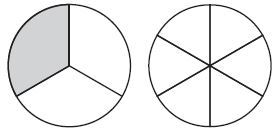Options:
a. $$\frac{1}{6}$$
b. $$\frac{2}{8}$$
c. $$\frac{2}{6}$$
d. $$\frac{2}{3}$$

Answer: $$\frac{2}{6}$$

Explanation: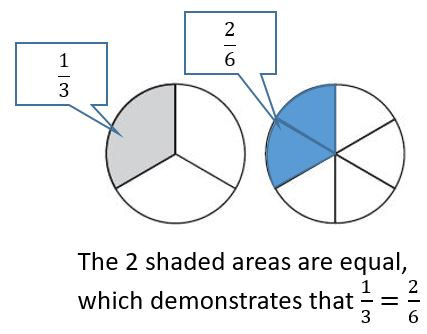$$\frac{1}{3}$$ = $$\frac{2}{6}$$
The correct answer is option C.

Spiral Review

Question 3.
Which number sentence is shown by the array?Options:
a. 8 − 2 = 6
b. 8 × 1 = 8
c. 2 + 8 = 10
d. 16 ÷ 2 = 8

Answer: 16 ÷ 2 = 8

Explanation:

Number of counters = 16
Number of rows = 2
Divide the Number of counters by the number of rows
= 16 ÷ 2 = 8
Thus the correct answer is option D.

Cody put 4 plates on the table. He put 1 apple on each plate. Which number sentence can be used to find the total number of apples on the table?
Options:
a. 4 + 1 = 5
b. 4 − 1 = 3
c. 4 × 1 = 4
d. 4 ÷ 2 = 2

Answer: 4 × 1 = 4

Explanation:

Cody put 4 plates on the table.
He put 1 apple on each plate.
The total number of apples on each table = 4 × 1 = 4
So, the correct answer is option C.

Question 5.
Which number sentence is a related fact to 7 × 3 = 21?
Options:
a. 7 + 3 = 10
b. 7 − 3 = 4
c. 7 × 2 = 14
d. 21 ÷ 3 = 7

Answer: 21 ÷ 3 = 7

Explanation:

The related division fact of 7 × 3 = 21 is 21 ÷ 3 = 7
The correct answer is option D.

Question 6.
Find the quotient.
4)$$\bar{3 6}$$
Options:
a. 9
b. 8
c. 7
d. 6

Explanation:

36 ÷ 4 = 9
4 divides 36 nine times. So the quotient is 9.
So, the correct answer is option A.

### Review/Test – Page No. 551

Question 1.
Alexa and Rose read books that have the same number of pages. Alexa’s book is divided into 8 equal chapters. Rose’s book is divided into 6 equal chapters. Each girl has read 3 chapters of her book.
Write a fraction to describe what part of the book each girl read. Then tell who read more pages. Explain.
Type below:
_____________

Explanation:

Given that,
Alexa and Rose read books that have the same number of pages.
Alexa’s book is divided into 8 equal chapters.
Rose’s book is divided into 6 equal chapters.
Each girl has read 3 chapters of her book.
The fraction of Alexa’s book = $$\frac{3}{8}$$
The fraction of Rose’s book = $$\frac{3}{6}$$
Now, compare the fractions to find who read more pages.
$$\frac{3}{8}$$ & $$\frac{3}{6}$$
The numerators of the two fractions are the same. So compare the denominators.
The denominator of the greater number will be the smallest fraction.
$$\frac{3}{8}$$ < $$\frac{3}{6}$$
By this, we can say that Rose read more pages than Alexa.

Question 2.
David, Maria, and Simone are shading same-sized index cards for a science project. David shaded $$\frac{2}{4}$$ of his index card. Maria shaded $$\frac{2}{8}$$ of her index card and Simone shaded $$\frac{2}{6}$$ of her index card.
For 2a–2d, choose Yes or No to indicate whether the comparisons are correct.
a. $$\frac{2}{4}$$ > $$\frac{2}{8}$$
i. yes
ii. no

Explanation:

$$\frac{2}{4}$$ > $$\frac{2}{8}$$
The denominators with the smallest number will be the greatest fraction.
Thus the statement $$\frac{2}{4}$$ > $$\frac{2}{8}$$ is true.

Question 2.
b. $$\frac{2}{8}$$ > $$\frac{2}{6}$$
i. yes
ii. no

Explanation:

$$\frac{2}{8}$$ > $$\frac{2}{6}$$
The numerators are same so compare the denominators.
The denominator with the greatest number will be the smallest fraction.
$$\frac{2}{8}$$ < $$\frac{2}{6}$$
Thus the statement is false.

Question 2.
c. $$\frac{2}{6}$$ < $$\frac{2}{4}$$
i. yes
ii. no

Explanation:

$$\frac{2}{6}$$, $$\frac{2}{4}$$
The numerators are same so compare the denominators.
The denominator with the greatest number will be the smallest fraction.
$$\frac{2}{6}$$ < $$\frac{2}{4}$$
Thus the statement is correct.

Question 2.
d. $$\frac{2}{8}$$ = $$\frac{2}{4}$$
i. yes
ii. no

Explanation:

$$\frac{2}{8}$$ = $$\frac{2}{4}$$
The numerators and denominators are different.
So, $$\frac{2}{8}$$ is not equal to $$\frac{2}{4}$$
The statement is false.

Go Math Grade 3 Chapter 9 Pdf Question 3.
Dan and Miguel are working on the same homework assignment. Dan has finished $$\frac{1}{4}$$ of the assignment. Miguel has finished $$\frac{3}{4}$$ of the assignment. Which statement is correct? Mark all that apply.
Options:
a. Miguel has completed the entire assignment.
b. Dan has not completed the entire assignment.
c. Miguel has finished more of the assignment than Dan.
d. Dan and Miguel have completed equal parts of the assignment.

Answer: B & C are the correct statements.

Explanation:

Given, Dan and Miguel are working on the same homework assignment.
Dan has finished $$\frac{1}{4}$$ of the assignment.
Miguel has finished $$\frac{3}{4}$$ of the assignment.
A. Miguel has completed the entire assignment.
Miguel has finished $$\frac{3}{4}$$ of the assignment. So the statement is false.
B. Dan has not completed the entire assignment.
Dan has finished $$\frac{1}{4}$$ of the assignment. So the statement is true.
C. Miguel has finished more of the assignment than Dan.
$$\frac{3}{4}$$ > $$\frac{1}{4}$$
So, the statement is true.
D. Dan and Miguel have completed equal parts of the assignment.
$$\frac{3}{4}$$ is not equal to $$\frac{1}{4}$$
Thus the statement is false.
So the correct answer is B & C.

### Review/Test – Page No. 552

Question 4.
Bryan cut two peaches that were the same size for lunch. He cut one peach into fourths and the other into sixths. Bryan ate $$\frac{3}{4}$$ of the first peach. His brother ate $$\frac{5}{6}$$ of the second peach. Who ate more peach? Explain the strategy you used to solve the problem.
___________

Explanation:

Given that,
Bryan cut two peaches that were the same size for lunch.
He cut one peach into fourths and the other into sixths.
Bryan ate $$\frac{3}{4}$$ of the first peach.
His brother ate $$\frac{5}{6}$$ of the second peach.
Compare the fractions $$\frac{3}{4}$$ and $$\frac{5}{6}$$
The numerators and denominators are different.
$$\frac{3}{4}$$ × $$\frac{6}{6}$$ = $$\frac{18}{24}$$
$$\frac{5}{6}$$ × $$\frac{3}{4}$$ = $$\frac{15}{24}$$
$$\frac{15}{24}$$ < $$\frac{18}{24}$$
By this we can say that Bryan’s brother ate more peach.

Question 5.
A nature center offers 2 guided walks. The morning walk is $$\frac{2}{3}$$ mile. The evening walk is $$\frac{3}{6}$$ mile. Which walk is shorter? Explain how you can use the model to find the answer.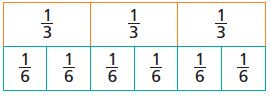___________

Explanation:

Given, The morning walk is $$\frac{2}{3}$$ mile
The evening walk is $$\frac{3}{6}$$ mile.
The shorter among both is $$\frac{3}{6}$$ i.e, evening walk$$\frac{2}{3}$$ > $$\frac{3}{6}$$

Question 6.
Chun lives $$\frac{3}{8}$$ mile from school. Gail lives $$\frac{5}{8}$$ mile from school. Use the fractions and symbols to show which distance is longer.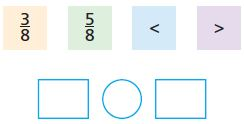$$\frac{□}{□}$$ mile

Answer: $$\frac{3}{8}$$ < $$\frac{5}{8}$$

Explanation:

Given,
Chun lives $$\frac{3}{8}$$ mile from school.
Gail lives $$\frac{5}{8}$$ mile from school.
Denominators are the same so we have to compare the numerators.
3 is less than 5. $$\frac{3}{8}$$ < $$\frac{5}{8}$$

### Review/Test – Page No. 553

Question 7.
Mrs. Reed baked four pans of lasagna for a family party. Use the rectangles to represent the pans.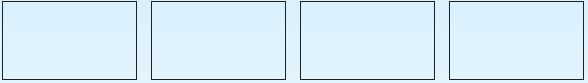Part A
Draw lines to show how Mrs. Reed could cut one pan of lasagna into thirds, one into fourths, one into sixths, and one into eighths.
Type below:
___________

Mrs. Reed could cut one pan of lasagna into thirds:Mrs. Reed could cut one pan of lasagna into fourths: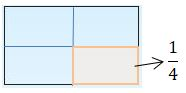Mrs. Reed could cut one pan of lasagna into sixths: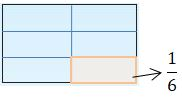Mrs. Reed could cut one pan of lasagna into eighths:Question 7.
Part B
At the end of the dinner, equivalent amounts of lasagna in two pans were left. Use the models to show the lasagna that might have been left over. Write two pairs of equivalent fractions to represent the models.
Type below:
___________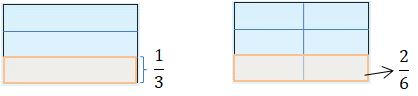$$\frac{1}{3}$$ = $$\frac{2}{6}$$$$\frac{1}{4}$$ = $$\frac{2}{8}$$

Question 8.
Tom rode his horse for $$\frac{4}{6}$$ mile. Liz rode her horse for an equal distance. What is an equivalent fraction that describes how far Liz rode? Use the models to show your work.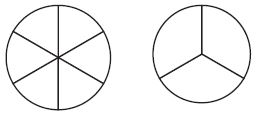$$\frac{4}{6}$$ = $$\frac{□}{□}$$

Answer: $$\frac{4}{6}$$ = $$\frac{2}{3}$$

Explanation:

The above figure shows that the fraction of the first figure $$\frac{4}{6}$$ is equal to the fraction of the second figure i.e., $$\frac{2}{3}$$.

Question 9.
Avery prepares 2 equal-size oranges for the bats at the zoo. One dish has $$\frac{3}{8}$$ of an orange. Another dish has $$\frac{1}{4}$$ of an orange. Which dish has more orange? Show your work. $$\frac{□}{□}$$

Answer: First, we need to find an equivalent fraction to $$\frac{1}{4}$$ so it would have the same denominator as $$\frac{3}{8}$$
$$\frac{1}{4}$$ = $$\frac{2}{8}$$ – equivalent fractions
Now we can compare the fractions:
$$\frac{2}{8}$$ < $$\frac{3}{8}$$
Therefore $$\frac{1}{4}$$ < $$\frac{3}{8}$$
So, the answer is $$\frac{3}{8}$$

### Review/Test – Page No. 554

Question 10.
Jenna painted $$\frac{1}{8}$$of one side of a fence. Mark painted $$\frac{1}{6}$$ of the other side of the same fence. Use >, =, or < to compare the parts that they painted.
$$\frac{1}{8}$$ ______ $$\frac{1}{6}$$

Answer: $$\frac{1}{8}$$ < $$\frac{1}{6}$$

Explanation:

Jenna painted $$\frac{1}{8}$$of one side of a fence.
Mark painted $$\frac{1}{6}$$ of the other side of the same fence.
The numerators of both fractions are the same. So compare the denominators.
$$\frac{1}{8}$$ & $$\frac{1}{6}$$
The denominator with the greatest number will be the smallest fraction.
So, $$\frac{1}{8}$$ < $$\frac{1}{6}$$

Question 11.
Bill used $$\frac{1}{3}$$ cup of raisins and $$\frac{2}{3}$$ cup of banana chips to make a snack. For 11a–11d, select True or False for each comparison.
a. $$\frac{1}{3}$$ > $$\frac{2}{3}$$
i. True
ii. False

Explanation:

The denominators are the same here. So check the numerators.
1 is less than 2.
$$\frac{1}{3}$$ > $$\frac{2}{3}$$
The statement is false.

Question 11.
b. $$\frac{2}{3}$$ = $$\frac{1}{3}$$
i. True
ii. False

Explanation:

The denominators and numerators are not equal in this equation.
So, the statement is false.

Question 11.
c. $$\frac{1}{3}$$ < $$\frac{2}{3}$$
i. True
ii. False

Explanation:

The denominators of both the fractions are the same.
Compare the numerators. 1 is less than 2.
So, $$\frac{1}{3}$$ < $$\frac{2}{3}$$.
The statement is true.

Question 11.
d. $$\frac{2}{3}$$ > $$\frac{1}{3}$$
i. True
ii. False

Explanation:

The denominators of both the fractions are the same.
Compare the numerators. 2 is greater than 1.
$$\frac{2}{3}$$ > $$\frac{1}{3}$$
The statement is true.

Question 12.
Jorge, Lynne, and Crosby meet at the playground. Jorge lives $$\frac{5}{6}$$ mile from the playground. Lynne lives $$\frac{4}{6}$$ mile from the playground. Crosby lives $$\frac{7}{8}$$ mile from the playground.
Part A
Who lives closer to the playground, Jorge or Lynne?
Explain how you know.
_____

Explanation:

Jorge lives $$\frac{5}{6}$$ mile from the playground.
Lynne lives $$\frac{4}{6}$$ mile from the playground.
The denominators are the same. So, compare the numerators.
5 is greater than 4. So, $$\frac{5}{6}$$ > $$\frac{4}{6}$$
Therefore, Lynne lives closer to the playground.

Question 12.
Part B
Who lives closer to the playground, Jorge or Crosby?
Explain how you know.
_____

Explanation:

Jorge lives $$\frac{5}{6}$$ mile from the playground.
Crosby lives $$\frac{7}{8}$$ mile from the playground.
Compare the fraction of both Jorge and Crosby.
$$\frac{5}{6}$$ × $$\frac{8}{8}$$ = $$\frac{40}{48}$$
$$\frac{7}{8}$$ × $$\frac{6}{6}$$ = $$\frac{42}{48}$$
$$\frac{40}{48}$$ < $$\frac{42}{48}$$
Therefore, Jorge lives closer to the playground.

### Review/Test – Page No. 555

Question 13.
Ming needs $$\frac{1}{2}$$ pint of red paint for an art project. He has 6 jars that have the following amounts of red paint in them. He wants to use only 1 jar of paint. Mark all of the jars of paints that Ming could use.
Options:
a. $$\frac{2}{3}$$ pint
b. $$\frac{1}{4}$$ pint
c. $$\frac{4}{6}$$ pint
d. $$\frac{3}{4}$$ pint
e. $$\frac{3}{8}$$ pint
f. $$\frac{2}{6}$$ pint

Answer: $$\frac{2}{3}$$ pint, $$\frac{3}{4}$$ pint, $$\frac{4}{6}$$ pint

Explanation:

We have to find all the jars that have an amount of paint greater than $$\frac{1}{2}$$
A. $$\frac{2}{3}$$ pint > $$\frac{1}{2}$$
B. $$\frac{1}{4}$$ pint < $$\frac{1}{2}$$
C. $$\frac{4}{6}$$ pint > $$\frac{1}{2}$$
D. $$\frac{3}{4}$$ pint < $$\frac{1}{2}$$
E. $$\frac{3}{8}$$ pint < $$\frac{1}{2}$$
F. $$\frac{2}{6}$$ pint < $$\frac{1}{2}$$

Question 14.
There are 12 people having lunch. Each person wants $$\frac{1}{3}$$ of a sub sandwich. How many whole sub sandwiches are needed? Use the models to show your answer.______ sub sandwiches

Explanation:

Given that, There are 12 people having lunch.
Each person wants $$\frac{1}{3}$$ of a sub sandwich.
Multiply the number of people with Each person wants of a sub sandwich.
12 × $$\frac{1}{3}$$
3 divides 12 four times. So, the answer is 4 sub sandwiches.

Question 15.
Mavis mixed $$\frac{2}{4}$$ quart of apple juice with $$\frac{1}{2}$$ quart of cranberry juice. Compare the fractions. Choose the symbol that makes the statement true.
$$\frac{2}{4}$$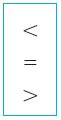$$\frac{1}{2}$$
______

Answer:  $$\frac{2}{4}$$ = $$\frac{1}{2}$$

Question 16.
Pat has three pieces of fabric that measure $$\frac{3}{6}$$, $$\frac{5}{6}$$, and $$\frac{2}{6}$$ yards long. Write the lengths in order from least to greatest.
Type below:
___________

Answer: $$\frac{2}{6}$$, $$\frac{3}{6}$$, $$\frac{5}{6}$$

Explanation:

The denominators of $$\frac{3}{6}$$, $$\frac{5}{6}$$, and $$\frac{2}{6}$$ are same. So, Compare the numerators 2 < 3, 5

### Review/Test – Page No. 556

Question 17.
Cora measures the heights of three plants. Draw a line to match each height on the left to the word on the right that describes its place in the order of heights.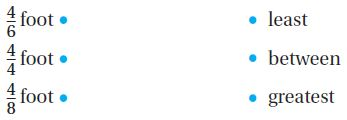Type below:
___________Explanation:

$$\frac{4}{6}$$ < $$\frac{4}{4}$$
$$\frac{4}{8}$$ < $$\frac{4}{4}$$
$$\frac{4}{6}$$ > $$\frac{4}{8}$$
The numerators are same. So, compare the denominators. The greatest fraction will have the lesser denominator.
$$\frac{4}{8}$$ < $$\frac{4}{6}$$ < $$\frac{4}{4}$$.

Question 18.
Danielle drew a model to show equivalent fractions.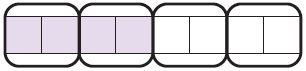Use the model to complete the number sentence.
$$\frac{1}{2}$$ = $$\frac{□}{□}$$ = $$\frac{□}{□}$$
Type below:
___________

Answer: $$\frac{1}{2}$$ = $$\frac{2}{4}$$ = $$\frac{4}{8}$$

Total number of boxes are 4 and each are grouped into 2 = 8
Out of 4 boxes 2 boxes are shaded = $$\frac{2}{4}$$
$$\frac{2}{4}$$ = $$\frac{1}{2}$$
Next out of 8 grouped squares 4 are shaded = $$\frac{4}{8}$$
$$\frac{4}{8}$$ = $$\frac{1}{2}$$
Therefore the equivalent fractions are $$\frac{1}{2}$$ = $$\frac{2}{4}$$ = $$\frac{4}{8}$$

Question 19.
Floyd caught a fish that weighed $$\frac{2}{3}$$ pound. Kira caught a fish that weighed $$\frac{7}{8}$$ pound. Whose fish weighed more? Explain the strategy you used to solve the problem.
_____

Explanation:

We need to find equivalent fractions with the same denominator:
So Make the denominators of $$\frac{2}{3}$$ and $$\frac{7}{8}$$ equal.
$$\frac{2}{3}$$ × $$\frac{8}{8}$$ = $$\frac{16}{24}$$
$$\frac{7}{8}$$ × $$\frac{3}{3}$$ = $$\frac{21}{24}$$
$$\frac{16}{24}$$ < $$\frac{21}{24}$$
Therefore $$\frac{2}{3}$$ < $$\frac{7}{8}$$

Question 20.
Sam went for a ride on a sailboat. The ride lasted $$\frac{3}{4}$$ hour.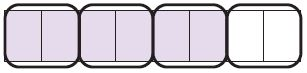What fraction is equivalent to $$\frac{3}{4}$$?
$$\frac{□}{□}$$

Answer: $$\frac{6}{8}$$

Explanation:

Total number of boxes are 4 and each is grouped into 2 = 8
Out of 4 boxes 3 boxes are shaded = $$\frac{3}{4}$$
Next out of 8 grouped squares 6 are shaded = $$\frac{6}{8}$$
Thus the fraction is equivalent to $$\frac{3}{4}$$ is $$\frac{6}{8}$$

Conclusion

Use the practice and Homework pages links to learn the concepts and skills which are provided in the Go Math Answer Key for Grade 3 Chapter 9 Compare Fractions. Help your child to climb greater heights and fall in love with Math learning. If you want to practice more problems you can go through the Go Math Grade 3 Answer Key Chapter 9 Compare Fractions Extra Practice.

Scroll to Top